# Sensitivity Factors from FORM method¶

Sensitivity Factors are evaluated under the following context: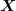denotes a random input vector, representing the sources of uncertainties,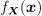its joint density probability,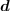a deterministic vector, representing the fixed variables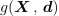the limit state function of the model,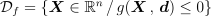the event considered here and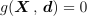its boundary (also called limit state surface).
The probability content of the event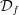is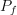:

(1)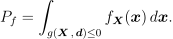In this context, the probabilitycan often be efficiently estimated by FORM or SORM approximations.
The FORM importance factors offer a way to analyze the sensitivity of the probability the realization of the event with respect to the parameters of the probability distribution of.
A sensitivity factor is defined as the derivative of the Hasofer-Lind reliability index with respect to the parameter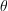. The parameteris a parameter in a distribution of the random vector.
If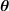represents the vector of all the parameters of the distribution ofwhich appear in the definition of the isoprobabilistic transformation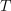, and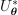the design point associated to the event considered in the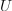-space, and if the mapping of the limit state function by theis noted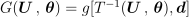, then the sensitivity factors vector is defined as: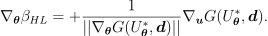The sensitivity factors indicate the importance on the Hasofer-Lind reliability index (refer to ) of the value of the parameters used to define the distribution of the random vector.

Here, the event considered is explicited directly from the limit state function: this is the classical structural reliability formulation. However, if the event is a threshold exceedance, it is useful to explicit the variable of interest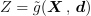, evaluated from the model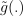. In that case, the event considered, associated to the threshold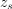has the formulation: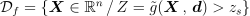and the limit state function is :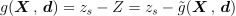.is the threshold exceedance probability, defined as: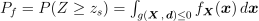. Thus, the FORM sensitivity factors offer a way to rank the importance of the parameters of the input components with respect to the threshold exceedance by the quantity of interest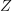. They can be seen as a specific sensitivity analysis technique dedicated to the quantity Z around a particular threshold rather than to its variance.